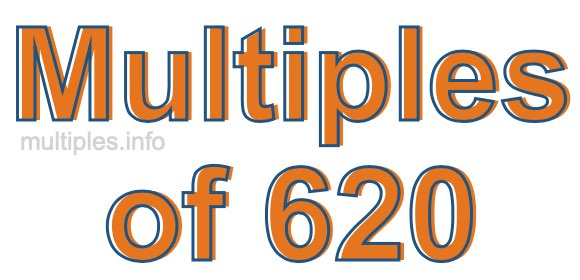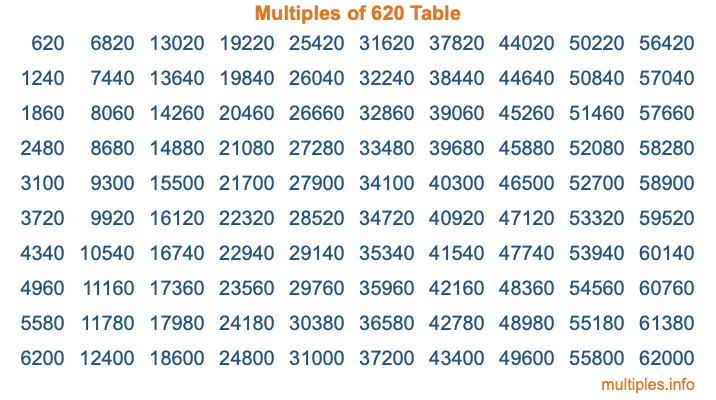Multiples of 620Welcome to the Multiples of 620 page. Here we will first teach you everything you will ever need to know about the multiples of 620, and then give you a study guide summary of everything we taught you to make sure you remember it all. Use this page to look up facts and learn information about the multiples of 620. This page will make you a multiples of six hundred twenty expert!

Definition of Multiples of 620
Multiples of 620 are all the numbers that when divided by 620 equal an integer. Each of the multiples of 620 are called a multiple. A multiple of 620 is created by multiplying 620 by an integer.

Therefore, to create a list of multiples of 620, you start with 1 multiplied by 620, then 2 multiplied by 620, then 3 multiplied by 620, and so on for as long as you want. Thus, the list of the first five multiples of 620 is 620, 1240, 1860, 2480, and 3100. To see a larger list of multiples of 620, see the printable image of Multiples of 620 further down on this page. We also have a category where you can choose any nth multiple of 620.

Multiples of 620 Checker
The Multiples of 620 Checker below checks to see if any number of your choice is a multiple of 620. In other words, it checks to see if there is any number (integer) that when multiplied by 620 will equal your number. To do that, we divide your number by 620. If the the quotient is an integer, then your number is a multiple of 620.

Is  a multiple of 620?

Least Common Multiple of 620 and ...
A Least Common Multiple (LCM) is the lowest multiple that two or more numbers have in common. This is also called the smallest common multiple or lowest common multiple and is useful to know when you are adding our subtracting fractions. Enter one or more numbers below (620 is already entered) to find the LCM.

Check out our LCM Calculator if you need more details about the Least Common Multiple or if you need the LCM for different numbers for adding and subtraction fractions.

nth Multiple of 620
As we stated above, 620 is the first multiple of 620, 1240 is the second multiple of 620, 1860 is the third multiple of 620, and so on. Enter a number below to find the nth multiple of 620.

th multiple of 620

Multiples of 620 vs Factors of 620
620 is a multiple of 620 and a factor of 620, but that is where the similarities end. All postive multiples of 620 are 620 or greater than 620. All positive factors of 620 are 620 or less than 620.

Below is the beginning list of multiples of 620 and the factors of 620 so you can compare:

Multiples of 620: 620, 1240, 1860, 2480, 3100, etc.

Factors of 620: 1, 2, 4, 5, 10, 20, 31, 62, 124, 155, 310, 620

As you can see, the multiples of 620 are all the numbers that you can divide by 620 to get a whole number. The factors of 620, on the other hand, are all the whole numbers that you can multiply by another whole number to get 620.

It's also interesting to note that if a number (x) is a factor of 620, then 620 will also be a multiple of that number (x).

Multiples of 620 vs Divisors of 620
The divisors of 620 are all the integers that 620 can be divided by evenly. Below is a list of the divisors of 620.

Divisors of 620: 1, 2, 4, 5, 10, 20, 31, 62, 124, 155, 310, 620

The interesting thing to note here is that if you take any multiple of 620 and divide it by a divisor of 620, you will see that the quotient is an integer.

Multiples of 620 Table
Below is an image of the first 100 multiples of 620 in a table. The table is in chronological order, column by column. The first column has the first ten multiples of 620, the second column has the next ten multiples of 620, and so on.The Multiples of 620 Table is also referred to as the 620 Times Table or Times Table of 620. You are welcome to print out our table for your studies.

Negative Multiples of 620
Although not often discussed or needed in math, it is worth mentioning that you can make a list of negative multiples of 620 by multiplying 620 by -1, then by -2, then by -3, and so on, to get the following list of negative multiples of 620:

-620, -1240, -1860, -2480, -3100, etc.

Multiples of 620 Summary
Below is a summary of important Multiples of 620 facts that we have discussed on this page. To retain the knowledge on this page, we recommend that you read through the summary and explain to yourself or a study partner why they hold true.

There are an infinite number of multiples of 620.

A multiple of 620 divided by 620 will equal a whole number.

620 divided by a factor of 620 equals a divisor of 620.

The nth multiple of 620 is n times 620.

The largest factor of 620 is equal to the first positive multiple of 620.

620 is a multiple of every factor of 620.

620 is a multiple of 620.

A multiple of 620 divided by a divisor of 620 equals an integer.

620 divided by a divisor of 620 equals a factor of 620.

Any integer times 620 will equal a multiple of 620.

Multiples of a Number
Here you can get the multiples of another number, all with the same attention to detail as we did for multiples of 620 on this page.

Multiples of
Multiples of 621
Did you find our page about multiples of six hundred twenty educational? Do you want more knowledge? Check out the multiples of the next number on our list!